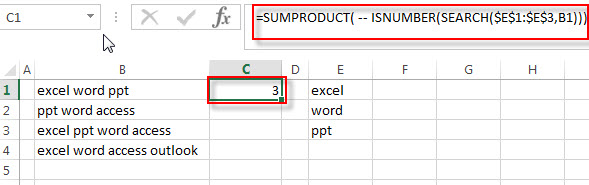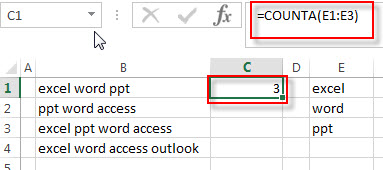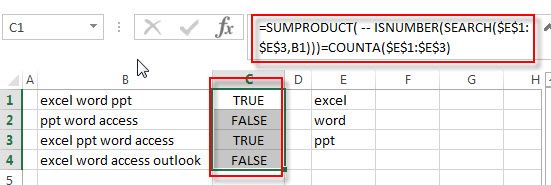# How to Check If Cell Contains All Values from Range

This post explains that how to check if a cell contains all of several values from a range in excel. How to check if cell contains all of many values in a list in excel 2013. How to check if all of values in a list can be found in a cell, if TRUE, then return TRUE, otherwise, returns FALSE.

## Check if Cell Contains all values from range

If you want to check if a cell contains all values in a list, you can use a combination of the SEARCH function, the SUMPRODUCT function, the ISNUMBER function and COUNTA function. And you need to create a new formula to count all matches at once, then compare to the total number of all values in list, if equal, we can know that that cell contains all values of list.

For example, assuming that you have a list of text strings in the range B1:B3, and you want to check if the Cell B1 contains all of values in another range E1:E3, you can write down the following formula:

`=SUMPRODUCT( -- ISNUMBER(SEARCH(\$E\$1:\$E\$3,B1)))=COUNTA(\$E\$1:\$E\$3)`

Let’s see how this formula works:

=SEARCH(\$E\$1:\$E\$3,B1)

The SEACH function returns position of the first character of find_text in a text string. And this formula will search each value from the range E1:E3 inside within_text in Cell B1, then returns position of each text string in Cell B1, so it will return an array result like this:

`{1;7;12}`

The returned result goes into the ISNUMBER function as its argument.

=ISNUMBER(SEARCH(\$E\$1:\$E\$3,B1))

The ISNUMBER function will check if a cell contains a numeric value, and this formula will check each items of array result returned by the SEACH function, if the item is a numeric value, then return TRUE, otherwise, returns FALSE. So it will return another array result like this:

`{TRUE;TRUE;TRUE}`

=– ISNUMBER(SEARCH(E1:E3,B1))

The double-dash is known as a double unary operator, it can convert the TRUE values to 1 and FALSE values to 0. So this formula returns an array of numbers like this:

`{1,1,1}`

=SUMPRODUCT( — ISNUMBER(SEARCH(\$E\$1:\$E\$3,B1)))The SUMPRODUCT returns a total sum of the array result returned by the ISNUMBER function with double-dash operator. So it returns 3.

= COUNTA(\$E\$1:\$E\$3)This formula returns the number of cells that are not empty in range E1:E3. It returns 3.

=SUMPRODUCT( — ISNUMBER(SEARCH(\$E\$1:\$E\$3,B1)))=COUNTA(\$E\$1:\$E\$3)If the result returned by the SUMPRODUCT is equal to the number of items in the range E1:E3, then we can know that all values in range E1:E3 can be found in Cell B1. The number of items of range E1:E3 can be got from the COUNTA function. So it returns TRUE.

### Related Formulas

• Check if Cell contains one of many values from range
Assuming that you have a list of text strings in the range B1:B3 and you want to check each text string if it contains one of several values in a range E1:E3. If it contains any of text string in range E1:E3, then it should be return TRUE value, otherwise, it should return FALSE…

### Related Functions

• Excel SEARCH function
The Excel SEARCH function returns the number of the starting location of a substring in a text string.The syntax of the SEARCH function is as below:= SEARCH  (find_text, within_text,[start_num])…
• Excel SUMPRODUCT function
The Excel SUMPRODUCT function multiplies corresponding components in the given one or more arrays or ranges, and returns the sum of those products.The syntax of the SUMPRODUCT function is as below:= SUMPRODUCT (array1,[array2],…)…
• Excel ISNUMBER function
The Excel ISNUMBER function returns TRUE if the value in a cell is a numeric value, otherwise it will return FALSE.The syntax of the ISNUMBER function is as below:= ISNUMBER (value)…
• Excel COUNTA function
The Excel COUNTA function counts the number of cells that are not empty in a range. The syntax of the COUNTA function is as below:= COUNTA(value1, [value2],…)…